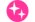Online ISSN : 2188-7977
Print ISSN : 0287-7651
ISSN-L : 0287-7651
ベイズ分析における点推定値例のRMSEとbiasによる比較――実験心理学の観点からの検討――

ジャーナル フリー 早期公開

この記事には本公開記事があります。The final version of this article is now available: Vol. 41 (2022), No. 1 pp. 1-7

Three types of point estimates of parameters, means, medians, and maximum a posteriori (MAP) estimates, were compared with respect to bias and root mean square error (RMSE). The overall results showed that the MAP estimates were the best among the three estimators for asymmetric posterior distributions of proportion parameters of binomial models, standard deviation parameters of univariate normal models, and correlation coefficient parameters of bivariate normal models. Although the comparisons were made for simple models, the results suggested that, in general, MAP estimates are appropriate for true values because MAP estimates are included in any highest-density intervals and because MAP estimates with flat prior distributions coincide with maximum likelihood estimates, which asymptotically converge to true values when sample sizes become large. A simple Python script to calculate a MAP estimate from Markov chain Monte Carlo (MCMC) sampling was presented.著者関連情報